The Rocscience International Conference 2021 Proceedings are now available. Read Now

# Generalized Hoek-Brown

The Generalized Hoek-Brown strength criterion is described by the following equation: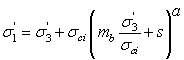where: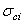is the uniaxial compressive strength (UCS) of the intact rock pieces

In most cases it is practically impossible to carry out triaxial or shear tests on rock masses at a scale which is necessary to obtain direct values of the mb, s and a parameters in the Generalized Hoek-Brown equation. Therefore some practical means of estimating the material constants mb, s and a is required. According to the latest research, the parameters of the Generalized Hoek-Brown criterion [ Hoek, Carranza-Torres & Corkum (2002) ], can be determined from the following equations: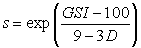where:

• GSI is the Geological Strength Index
• mi is a material constant for the intact rock
• the parameter D is a "disturbance factor" which depends upon the degree of disturbance to which the rock mass has been subjected by blast damage and stress relaxation. It varies from 0 for undisturbed in situ rock masses to 1 for very disturbed rock masses.

Consequently, the input for the Generalized Hoek-Brown criterion in Slide2 offers two options:

• Define strength envelope using mb, s, a
• Define strength envelope using GSI, mi, D

Define Strength using mb, s, a

If this option is selected, then you can enter the parameters mb, s and a directly.

NOTE: the parameters mb, s and a can be calculated from GSI, mi and D using the above equations, by selecting the GSI button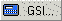in the Define Material Properties dialog. See the Parameter Calculator topic for more information.

Define Strength using GSI, mi, D

If this option is selected, then you can enter the parameters GSI, mi and D directly.

NOTE: if you select the Pick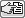button beside an edit box, you may access interactive tables and charts for:

• the estimation of mi based on rock type
• the estimation of GSI based on rock structure and surface conditions
• the estimation of Disturbance Factor based on excavation type and techniques
• the estimation of Intact Uniaxial Compressive Strength (UCS) based on field estimates

Select the Pick button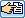for the parameter that you wish to estimate, and you will see a table or chart which allows you to select an appropriate value for the parameter, for your material.

Parameters vary with depth

If you are defining strength using the GSI,mi,D option, then you will have the additional capability of defining these parameters as a function of depth. To do this:

1. Select the Parameters vary with depth checkbox and select the Define button.
2. You will see another dialog which allows you to define the parameters GSI, mi, D as Constant, F(Depth) or F(Datum) by selecting from the Type drop-list and entering the following.

Constant

GSI, mi or D is constant throughout the material.

F(Depth)

GSI, mi or D is a function of depth, where depth is measured from the top of the material layer, to the center of a slice base. For example:

• GSI (Top) is the value of GSI at the top of the material layer.
• GSI Change is the rate of change of GSI with depth.
• The Cutoff checkbox allows you to specify a limiting maximum (or minimum) value of GSI. If the rate of GSI Change is negative, then the Cutoff value represents the minimum GSI.

F(Datum)

GSI, mi or D is a function of depth, where depth is measured from a user specified Datum (y-coordinate) to the center of a slice base. For example:

• GSI (Datum) is the value of GSI at the Datum elevation.
• GSI Change is the rate of change of GSI with vertical distance (ydatum-y) from the Datum.
• The Cutoff checkbox allows you to specify a limiting maximum (or minimum) value of GSI. If the rate of GSI Change is negative, then the Cutoff value represents the minimum GSI.
• Datum is the datum elevation (y-coordinate).

UCS (intact)

The intact UCS (ultimate compressive strength) is required input for the Generalized Hoek-Brown criterion, regardless of which method you have chosen to define the strength envelope (mb,s,a or GSI,mi,D).